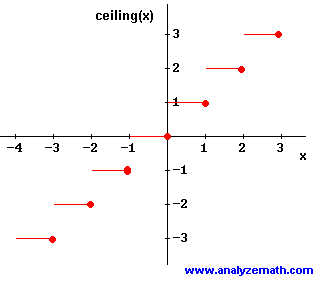# Ceiling Function

## Definition of the Ceiling Function

The ceiling function ceiling(x) is defined as the function that outputs the smallest integer greater than or equal to x. Below is shown the graph of ceiling(x)The domain of ceiling(x) is the set of all real numbers. The range of ceiling(x) is the set of all integers.

### Example

Evaluate ceiling(x) for various values of x.
ceiling(1.5) = 2
ceiling(0) = 0
ceiling(2) = 2
ceiling(- 3) = - 3
ceiling(- 1.5) = - 1